1. Support Center
2. Knowledge Base
3. Document Type Configuration

# Learn how to use Verifiers

## How to use verifiers to filter out false positive results

Verifiers are one of the rule-based validation approaches, which filter out the false-positives. These let the user narrow down extraction results by applying some additional logic and checks to the extraction results. One can for example say that a specific type of information will always occur on the first page of the document, or that it must be on the lower half of the page, etc.
The verifiers will be processed after the transformers.
The verifiers with a star symbol * follow in the next release.
 Verifier Description coords: Position Range Rejects candidates which are not with the rectangle made of `left`, `right`, `top`, `bottom` coordinates, e.g. at the top half page. coords: Position Range Center Rejects candidates whose centers are not with the rectangle made of `left`, `right`, `top`, `bottom` coordinates, e.g. at the top half page. image: aspect ratio Restricts the results to a range of aspect ratio key-value: Pair Orientation* Defintion of keywords, which should be found in the near (horizontaly or verticaly) of the candidate number: Page Number Checks whether a candidate is on the specified page. Candidates found on other pages are ignored. number: Value Range Can only be used if the `output_data_type` is numeric (integer or float). Candidates with a value that is smaller than `minimum value` or larger than `maximum_value` are rejected. pick_nth This verifier picks the n-th element of the sorted candidate list string: Ends With Candidates that do not end with the specific string are rejected. string: Has Not Pattern Candidates containing the specified `pattern` are rejected. string: Has Pattern Candidates that do not contain the specified `pattern` are rejected. In this case only Swiss vat numbers are allowed string: Starts Not With Skips candidates whose prediction value start with a specific string. string: Starts With Candidates that do not start with the specific string are rejected. string: String Length Rejects candidates that do not have the right number of "characters"

### Verifier "coords: Position Range"

Definition of coordinates, where the candidate have to be found. The verifier can restrict the result horizontally (left coordinate [0..1] (float) - right coordinate [0..1] (float)) and vertically (top coordinate [0..1] (float) - bottom coordinate [0..1] (float)). All values are defined in percent between 0 and 1, using a point as decimal.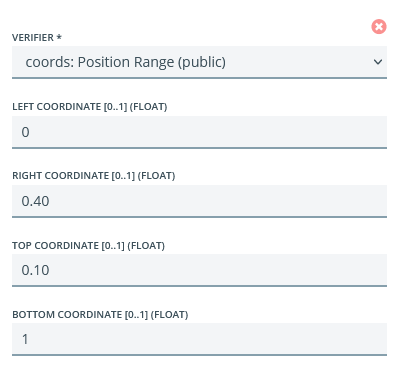Parameter Description Left coordinate Left coordinate of the rectangle (related to page width) 0 <= value <= 1 Right coordinate Right coordinate of the rectangle (related to page width) 0 <= value <= 1 Top coordinate Top coordinate of the rectangle (related to page height) 0 <= value <= 1 Bottom coordinate Bottom coordinate of the rectangle (related to page height) 0 <= value <= 1

### Verifier "coords: Position Range Center"

Definition of coordinates, where the center of the candidate have to be found. The verifier can restrict the result horizontally (left coordinate [0..1] (float) - right coordinate [0..1] (float)) and vertically (top coordinate [0..1] (float) - bottom coordinate [0..1] (float)). All values are defined in percent between 0 and 1, using a point as decimal.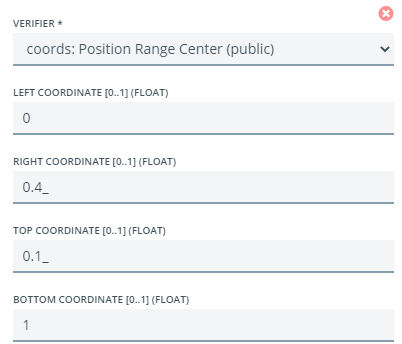Parameter Description Left coordinate Left coordinate of the rectangle (related to page width) 0 <= value <= 1 Right coordinate Right coordinate of the rectangle (related to page width) 0 <= value <= 1 Top coordinate Top coordinate of the rectangle (related to page height) 0 <= value <= 1 Bottom coordinate Bottom coordinate of the rectangle (related to page height) 0 <= value <= 1

### Verifier "key-value: Pair Orientation"

Definition of keywords, which should be found in the near (horizontaly or verticaly) of the candidate.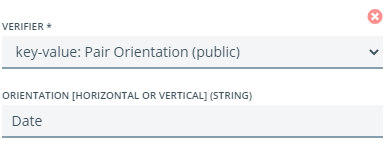Parameter Description Orientation (string) Search string (regex)

### Verifier "image: Aspect ratio"

Check the aspect ratio of the result tokens (length / height).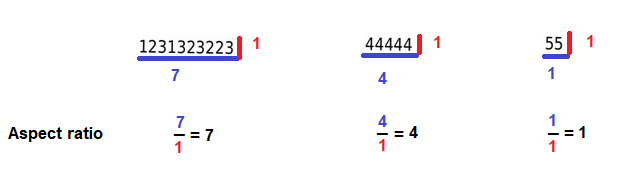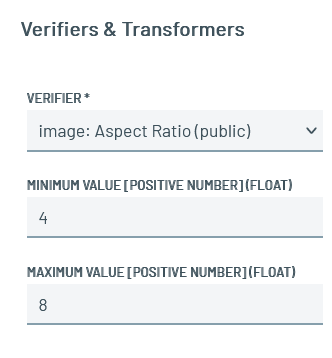Parameter Description Minimum value minimum ratio Maximum value maximum ratio

### Verifier "number: Page Number"

Checks whether a candidate is on the specified page. Candidates found on other pages are ignored. Here an example to extract the invoice date, which is normaly placed on the first page of an invoice.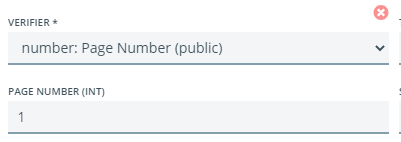Parameter Description Page number Page number 1 = first page

### Verifier "number: Value Range"

Can only be used if the output_data_type is numeric (integer or float). Candidates with a value that is smaller than minimum value or larger than maximum_value are rejected. Here an example to determine percentage values ( 0<= value <= 100).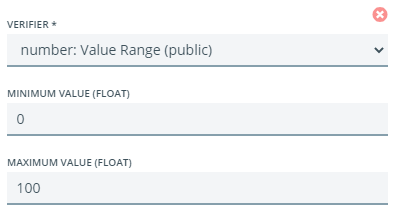Parameter Description Minimum value Minimal value Maximum value Maximal value

### Verifier "pick_nth"

This verifier picks the n-th element of the sorted candidate list, in this example the second element (zero-based index).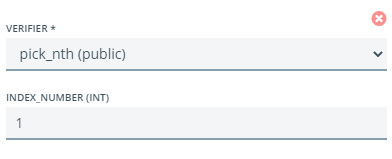Parameter Description Index number Index of the value 0 = first value
Result before restriction: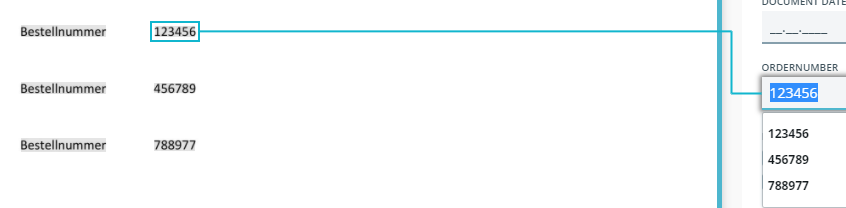Result after restriction: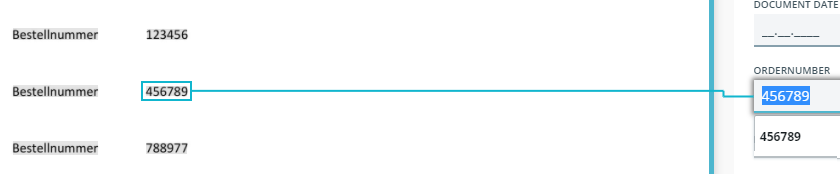### Verifier "string: Ends With"

Candidates that do not end with the specific string are rejected. As example to restrict candidates to amounts with to decimal places.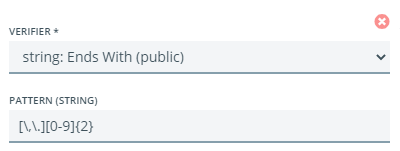Parameter Description Pattern (string) Search string (regex)

### Verifier "string: Has Not Pattern"

Candidates containing the specified pattern are rejected. As example that the result is no German IBAN number.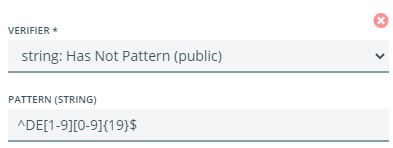Parameter Description Pattern (string) Search string (regex)

### Verifier "string: Has Pattern"

Candidates that do not contain the specified pattern are rejected. In this case only Swiss vat numbers are allowed.
 Parameter Description Pattern (string) Search string (regex)

### Verifier "string: Starts Not With"

Skips candidates whose prediction value start with a specific string. In this case remove all tax identification number candidates with German identifier.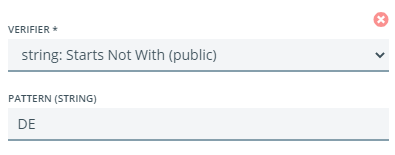Parameter Description Pattern (string) Search string (regex)

### Verifier "string: Starts With"

Skips candidates whose prediction value not start with a specific string. In this case remove all tax identification number candidates, except Austrian ids.Parameter Description Pattern (string) Search string (regex)

### Verifier "string: String Length"

Rejects candidates that do not have the right number of "characters".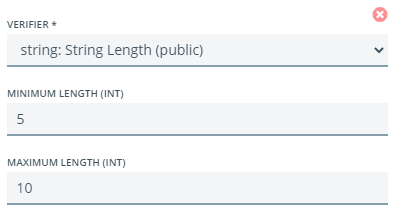Parameter Description Minimum length minimal length of the value Maximum length maximal length of the value